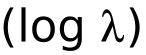# Lisplog

Blogging in Lisp

Search

## Going Supersonic in Elon Musk's Test Hyperloop Tube

Submitted by Bill St. Clair on Mon, 28 Aug 2017 12:43:10 GMT

Elon Musk tweeted that it "Might be possible to go supersonic in our test Hyperloop tube, even though it's only 0.8 miles long." This post computes the acceleration necessary for that.

Speed of sound: 767 mph = 1125 feet/second
1/2 test track length = 0.4 * 5280 feet = 2112 feet

t = time in seconds
a = acceleration in feet/second

1. 1/2 * a * t^2 = 2112 (distance from acceleration and time)
2. t * a = 1125 (speed from acceleration and time)

3. t = 1125 / a (divide both sides of 2 by a)
4. 1/2 * a * (1125/a)^2 = 2112 (plug 3 into 1)
5. 1/2 * a * 1,265,625 / a^2 = 2112 (do the square in 4)
6. 632812.5 / a = 2112 (do the arithmetic in 5)
7. a = 632812.5 / 2112 (multiply both sides of 6 by a and divide by 2112)
8. a = 299.6 feet/second
9. g = 32 feet/second^2 (acceleration due to gravity)
10. a = 299.6 / 32 = 9.4 g (divide 8 by (32 feet/second)/g)
11. t = 2 * 1125 / a = 7.6 seconds (time to accelerate AND decelerate)

So a Hyperloop vehicle could accelerate to the speed of sound and decelerate back to a standstill in 0.8 miles by accelerating at 9.4 g for 3.8 seconds and then decelerating at 9.4 g for 3.8 seconds.

Wikipedia's G-force page says that untrained humans can survive 10 g horizontal, eyes in or out, for 1 minute, so it's survivable.